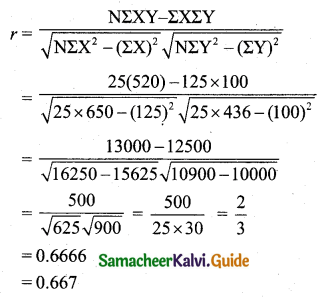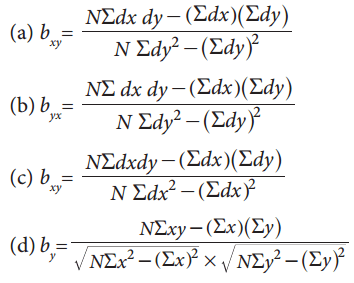Tamilnadu State Board New Syllabus Samcheer Kalvi 11th Business Maths Guide Pdf Chapter 9 Correlation and Regression Analysis Ex 9.3 Text Book Back Questions and Answers, Notes.

## Tamilnadu Samacheer Kalvi 11th Business Maths Solutions Chapter 9 Correlation and Regression Analysis Ex 9.3

### Samacheer Kalvi 11th Business Maths Correlation and Regression Analysis Ex 9.3 Text Book Back Questions and Answers

Question 1.
An example of a positive correlation is:
(a) Income and expenditure
(b) Price and demand
(c) Repayment period and EMI
(d) Weight and Income
(a) Income and expenditure

Question 2.
If the values of two variables move in the same direction then the correlation is said to be:
(a) Negative
(b) Positive
(c) Perfect positive
(d) No correlation
(b) PositiveQuestion 3.
If the values of two variables move in the opposite direction then the correlation is said to be:
(a) Negative
(b) Positive
(c) Perfect positive
(d) No correlation
(a) Negative

Question 4.
Correlation co-efficient lies between:
(a) 0 to ∞
(b) -1 to +1
(c) -1 to 0
(d) -1 to ∞
(b) -1 to +1Question 5.
If r(X, Y) = 0 the variables X and Y are said to be:
(a) Positive correlation
(b) Negative correlation
(c) No correlation
(d) Perfect positive correlation
(c) No correlation

Question 6.
The correlation coefficient from the following data N = 25, ΣX = 125, ΣY = 100, ΣX2 = 650, ΣY2= 436, ΣXY = 520:
(a) 0.667
(b) -0.006
(c) -0.667
(d) 0.70
(a) 0.667
Hint:Question 7.
From the following data, N = 11, ΣX = 117, ΣY = 260, ΣX2 = 1313, ΣY2 = 6580, ΣXY = 2827. the correlation coefficient is:
(a) 0.3566
(b) -0.3566
(c) 0
(d) 0.4566
(a) 0.3566
Hint:Question 8.
The correlation coefficient is:(b) r(X, Y) = $$\frac{cov(x, y)}{\sigma_{x} \sigma_{y}}$$Question 9.
The variable whose value is influenced or is to be predicted is called:
(a) dependent variable
(b) independent variable
(c) regressor
(d) explanatory variable
(a) dependent variable

Question 10.
The variable which influences the values or is used for prediction is called:
(a) Dependent variable
(b) Independent variable
(c) Explained variable
(d) Regressed
(b) Independent variableQuestion 11.
The correlation coefficient:(a) $$r=\pm \sqrt{b_{x y} \times b_{y x}}$$

Question 12.
The regression coefficient of X on Y:(a) $$b_{x y}=\frac{\mathrm{N} \Sigma d x d y-(\Sigma d x)(\Sigma d y)}{\mathrm{N} \Sigma d y^{2}-(\Sigma d y)^{2}}$$Question 13.
The regression coefficient of Y on X:(c) $$b_{y x}=\frac{\mathrm{N\Sigma} d x d y-(\Sigma d x)(\Sigma d y)}{\mathrm{N} \Sigma d x^{2}-(\Sigma d x)^{2}}$$

Question 14.
When one regression coefficient is negative, the other would be:
(a) Negative
(b) Positive
(c) Zero
(d) None of these
(a) NegativeQuestion 15.
If X and Y are two variates, there can be at most:
(a) one regression line
(b) two regression lines
(c) three regression lines
(d) more regression lines
(b) two regression lines

Question 16.
The lines of regression of X on Y estimates:
(a) X for a given value of Y
(b) Y for a given value of X
(c) X from Y and Y from X
(d) none of these
(a) X for a given value of YQuestion 17.
Scatter diagram of the variate values (X, Y) give the idea about:
(a) functional relationship
(b) regression model
(c) distribution of errors
(d) no relation
(a) functional relationship

Question 18.
If regression co-efficient of Y on X is 2, then the regression co-efficient of X on Y is:
(a) ≤ $$\frac{1}{2}$$
(b) 2
(c) > $$\frac{1}{2}$$
(d) 1
(a) ≤ $$\frac{1}{2}$$Question 19.
If two variables move in a decreasing direction then the correlation is:
(a) positive
(b) negative
(c) perfect negative
(d) no correlation
(a) positive

Question 20.
The person suggested a mathematical method for measuring the magnitude of the linear relationship between two variables say X and Y is:
(a) Karl Pearson
(b) Spearman
(c) Croxton and Cowden
(d) Ya Lun Chou
(a) Karl PearsonQuestion 21.
The lines of regression intersect at the point:
(a) (X, Y)
(b) $$(\overline{\mathrm{X}}, \overline{\mathrm{Y}})$$
(c) (0, 0)
(d) (σx, σy)
(b) $$(\overline{\mathrm{X}}, \overline{\mathrm{Y}})$$

Question 22.
The term regression was introduced by:
(a) R.A Fisher
(b) Sir Francis Galton
(c) Karl Pearson
(d) Croxton and Cowden
(b) Sir Francis GaltonQuestion 23.
If r = -1, then correlation between the variables:
(a) perfect positive
(b) perfect negative
(c) negative
(d) no correlation
(b) perfect negative

Question 24.
The coefficient of correlation describes:
(a) the magnitude and direction
(b) only magnitude
(c) only direction
(d) no magnitude and no directionIf Cov(x, y) = -16.5, $$\sigma_{x}^{2}$$ = 2.89, $$\sigma_{y}^{2}$$ = 100. Find correlation coeffient.